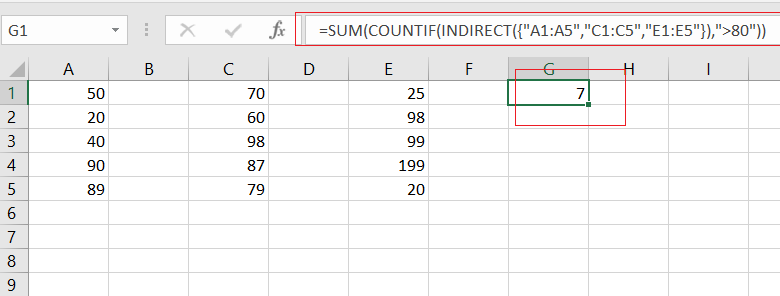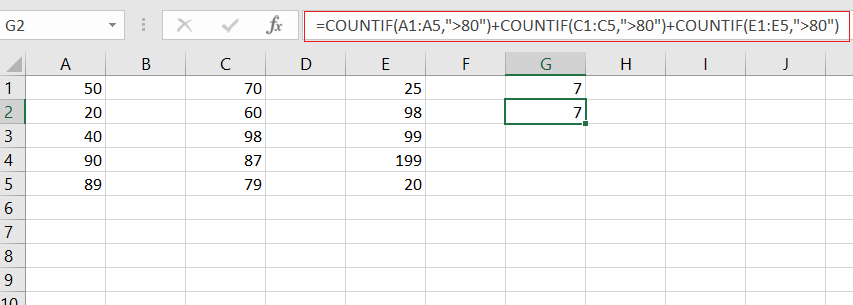# Count Non-Contiguous Range using COUNTIF

This post will guide you how to count a non-contiguous range with criteria in COUNTIF function in Excel 2013/2016 or Excel office 365. How do I build a formula for counting non-contiguous range in Excel using COUNTIF function in Excel.

## What is Non-Contiguous Range?

A non-contiguous range consists of tow of more separate ranges in your current worksheet. And this post will show you one formula that counting the non-contiguous range with criteria in Excel.

## Generic Formula

If you want to count a non-contiguous range with criteria, and you can use the COUNTIF function in combination with the INDIRECT and the SUM function. The general formula is like this:

=SUM(COUNTIF(INDIRECT({“range1″,”range2″,”range3”}),criteria))

The syntax of Explanations is as below:

• SUM – the sum function can be used to add up values in the given cells.
• COUNTIF – COUNT the number of cells that match a certain condition.
• INDIRECT – return a valid cell reference from a given text string.

## COUNT Non-Contiguous Range Using COUNTIF

Assuming you have tree ranges A1:A5, C1:C5, and E1:E5 in your worksheet and you wish to count the number of values that is greater than 80. And you can use the below formula:

=SUM(COUNTIF(INDIRECT({“A1:A5″,”C1:C5″,”E1:E5″}),”>80″))Let’s See That How This Formula Works:

The COUNTIF function can only count the number of cells in a range that match a given condition in Excel. And if you want to use the COUNTIF function with non-contiguous ranges, and you will get an error value. The best method is that you can use the INDIRECT function to get a valid cell reference from an array list that contain multiple text string.

The multiple ranges that returned by the INDIRECT function are passed into COUNTIF function. As the COUNTIF function receives an array list, it will return an array result also.

Finally, you can use the SUM function to add up all items in an array.

You can also use another solution to count Non-contiguous range using COUNTIF function. And using more that one COUNTIF function to count the numbers. The formula is like this:

=COUNTIF(A1:A5,”>80″)+COUNTIF(C1:C5,”>80″)+COUNTIF(E1:E5,”>80″)### Related Functions

• Excel SUM function
The Excel SUM function will adds all numbers in a range of cells and returns the sum of these values. You can add individual values, cell references or ranges in excel.The syntax of the SUM function is as below:= SUM(number1,[number2],…)…
• Excel COUNTIF function
The Excel COUNTIF function will count the number of cells in a range that meet a given criteria. This function can be used to count the different kinds of cells with number, date, text values, blank, non-blanks, or containing specific characters.etc.= COUNTIF (range, criteria)…
• Excel INDIRECT function
The Excel INDIRECT function returns the cell reference based on a text string, such as: type the text string “A2” in B1 cell, it just a text string, so you can use INDIRECT function to convert text string as cell reference….

Related Posts

6 Best Free Wedding Budget Templates

This post will show you some free wedding budget spreadsheet templates for both google sheets and Microsoft Excel Spreadsheet, as well as some explanations of the features or important function points of these templates. You can then easily make modifications ...

Calculate Cumulative Totals with Excel SUM Function

Today, through a simple example, we will show you how to use one of the most common-used Mathematical functions in excel, the SUM function, to add up the sum. In our daily life, we keep an account of what we ...

Check Dates in chronological order

Assume you have a date list that has different date formats, as seen in the accompanying picture. In this instance, Excel's Sort function will fail to sort them appropriately. However, you may convert all various date formats to a particular ...

How to Use 3D SUM Multiple Worksheets

To sum a range of numbers is straightforward for most Excel users, but do you know how to establish a 3D reference to total the same range of numerous sheets. In this post, I will present the steps for this ...

If Cell Begins with One of Three Supplied Characters

If you want to test values to see if they begin with some given specific characters like “x”, ”y”, or “z”, you can create a formula with COUNTIF and SUM functions to return results. EXAMPLE You can see “TRUE” or ...

Average per Week by Formula in Excel

We usually apply AVERAGE function or relevant functions to return average directly in Excel worksheet. But in some situations, only applying average relevant functions cannot figure out our problem. Sometimes we can create a formula with functions and mathematical operation ...

How to Sum if Contains an Asterisk

To add numbers together we need to apply SUM function. And if we want to add numbers based on some conditions, we can add criteria with the help of SUMIFS function, SUMIFS can filter data with multiple criteria effectively. If ...

How to Sum in Vertical Range

If we want to add numbers based on some conditions in Excel worksheet, we can add criteria with the help of SUMIFS function, SUMIFS can filter data with multiple criteria effectively. In this article, we will introduce you the method ...

How to Sum in Horizontal Range

To add numbers together we need to apply SUM function. But if we want to add numbers based on some conditions, we can add criteria with the help of SUMIFS function, SUMIFS can filter data with multiple criteria effectively. In ...

How to Sum with Criteria and Or Logic in Excel

To add numbers together we need to apply SUM function. And if we want to add numbers based on some conditions, we can add criteria with the help of SUMIFS function, SUMIFS can filter data with multiple criteria effectively. If ...

Sidebar